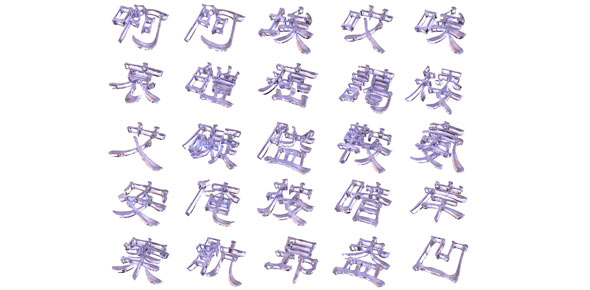# Mda Mid

34 | Total Attempts: 76SettingsÕżÜń®║µĀ╝ńÜäķĪīńø«Ķ½ŗõ╗źķĆŚķ╗×+ń®║ńÖĮķŹĄķÜöķ¢ŗ’╝īõĖ”õŠØńģ¦ĶĆāÕÅżńÜäķĀåÕ ║ÅÕĪ½Õ»½ŃĆéµĖ¼ķ®Śµ£ĆÕŠīµ£āµ£ēĶ¦ŻńŁö’╝īõĮåµś»õ╗¢µ▓ÆķéŻķ║╝Ķü░µśÄ µēĆõ╗źµ£ēõ║øķī»ńÜäÕÅ»ĶāĮõĮĀÕ»½Õ░Ź’╝īĶ½ŗĶć¬ÕĘ▒ńĢÖµäÅõĖĆõĖŗ

Related Topics
• 1.
The objective of _____________________ is to avoid the use of only a single variable to represent a concept, and instead to use several variables as indicators, all representing differing facets of the concept to obtain a more ‘’well-rounded’’ perspective.
• 2.
If the single dependent variable is metric, then the appropriate technique is _______.
• 3.
If a set of dependent/independent variable relationships is postulated, then _______ is appropriate.
• 4.
If the single dependent variable is metric and the independent variables are nonmetric _______ should be selected.
• 5.
If the several dependent variables are metric and the independent variables are nonmetric, _______ should be selected.
• 6.
_______ is the degree to which a measure accurately represents what it is supposed to.
• 7.
There are two basic kind of data: nonmetric and metric. _______ measurements can be made with either an interval or a _______scale
• 8.
If the single dependent variable is nonmetric, then the appropriate technique are _______ and linear probability models.
• 9.
Four common approaches for dealing with missing data include use of observations with ______________, _____________________, ______________, and ______________ procedure.
• 10.
_______ and _____________________ are tools that use to examine the shape of the distribution of a single variable.
• 11.
If a nonlinear relationship is detected, ______________ and _____________________ can be used to remedy the situation.
• 12.
_______ is the degree to which the observed variable measures the ’’true ’’ value and is “error free”.
• 13.
_____________________ model is most appropriate when the primary objective is about prediction or the minimum number of factors needed to account for the maximum portion of the variance represented in the original set of variables, and when prior knowledge suggests that specific and error variance represent a relatively small proportion of the total variance.
• 14.
The most problematic issue in independent variable selection is _______ which concerns the inclusion of irrelevant variables or the omission of relevant variables from the set of independent variables.
• 15.
The impact of _______ is to reduce any single independent variable’s predictive power by the extent to which it is associated with the other independent variables.
• 16.
_______ is indicator of the effect that the other independent variables have on the standard error of a regression coefficient.
• 17.
The most common remedy for correlated errors is the _____________________ analysis that represents the omitted factor.
• 18.
A more reliable approach to test for normality is the _____________________, which compares the cumulative distribution of actual data values with the cumulative distribution of a normal distribution.
• 19.
Five criteria are usually utilized to decide on the number of factors to extract. They include _____________________, ______________, _____________________, ______________, and _____________________.
• 20.
The _____________________ is most appropriate when the primary objective is to identify the latent dimensions or constructs represented in the original variables, and the researcher has little knowledge about the amount of specific and error variance and therefore wishes to eliminate this variance.
• 21.
When we select among the three data reduction options, ______________ or ______________ are more appropriate if generalizability and transferability is desired. If the ______________ is a well-constructed, valid, and reliable instrument, then it is probably the best alternative.
• 22.
Two measures to quantify the degree of intercorrelations among the variables and the appropriateness of factor analysis are the _____________________ and _____________________.
• 23.
Regarding the sample size question, the researcher generally would not factor analyze a sample of fewer than 50 observations, and preferably the sample size should be 100 or large. As a general rule, the minimum is to have at least five times as many observations as the number of variables to be analyzed, and the more acceptable sample size would have a _______ ratio.
• 24.
There are several approaches to assist the researcher in finding the best regression model. The _____________________ computes a regression equation with all the independent variables, and then deletes independent variables that do not contribute significantly.
• 25.
To test the hypothesis that the amount of variation explained by the regression model is more than the variation explained by the average, the _______ is used.
• 26.
___ is the probability of incorrectly rejecting the null hypothesis
• 27.
______________ are correlations between the original variables and the factors, and the key to understanding the nature of a particular factor.
• 28.
Heteroscedastic variables can be remedied through _____________________.
• 29.
Power in multiple regression refers to the probability of detecting as statistical significant a specific level of ___ or a ______________ at a specified significance level for a specific sample size.
• 30.
Two of the more common measures for assessing both pairwise and multiple variable collinearity are (1)_______ and (2)its inverse-_______.
• 31.
There are two basic kinds of data nonmetric and metric. Nonmetric measurements can be made with either a nominal or an _______ scale.
• 32.
Power is determined by _______, _______, and _______.
• 33.
Linearity is a critical issue in regression analysis, it can be easily examined through ______________ or _____________________.
• 34.
_______ is an observation that is substantially different from the other observations on one or more characteristics.